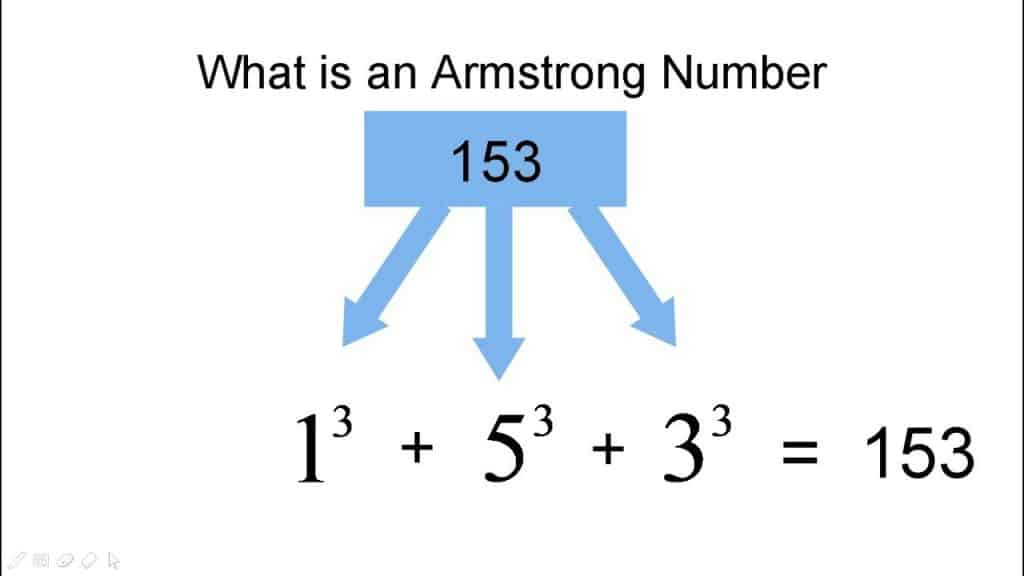# Armstrong Number In C Programming Language

Armstrong Number In C is the number that is equal to the sum of all digits to the power of the total number of digits in that number. To understand better check the example given below.

In number theory, a narcissistic number, an Armstrong number & it is named after Michael F. Armstrong is a number that is the sum of its own digits each raised to the power of the number of digits.Example 1: The Number 153 which is an Armstrong number

Let’s Prove that this number is Armstrong number:

Total digits in number = 3

1^3 = 1*1*1 = 1

5^3 = 5*5*5 = 125

3^3 = 3*3*3 = 9

S0, 153 = (1*1*1)+(5*5*5)+(3*3*3)

153= 1+125+27 , Hence Proved

Example 2: The Number 370 is an Armstrong number.

Let’s prove that this number is Armstrong number:

Total digits in number = 3

3^3 = 3*3*3 = 27

7^3 = 7*7*7 = 343

0^3 = 0*0*0 = 0

S0, 370 = (3*3*3)+(7*7*7)+(0*0*0)

370= 27+343+0 , hence proved

## Armstrong Number In C Program Code:

```#include<stdio.h>
int main()
{
int num,r,sum=0,temp;
printf("enter the number=");
scanf("%d",&num);
temp=num;
while(num>0)
{
r=num%10;
sum=sum+(r*r*r);
num=num/10;
}
if(temp==sum)
printf("armstrong  number ");
else
printf("not armstrong number");
return 0;
}```

Also Check: Armstrong Number In Java

Try Best Ide For C programming Language is Turbo C++. Still having difficulty understanding, then you can watch the below video to understand the Armstrong number program:

Few easy & quick tricks explained in this video & you can implement them easily as well.

### Conclusion:

You can check the source code of this program in this article itself. Feel free to contact us if you have any questions regarding this program or anything else.